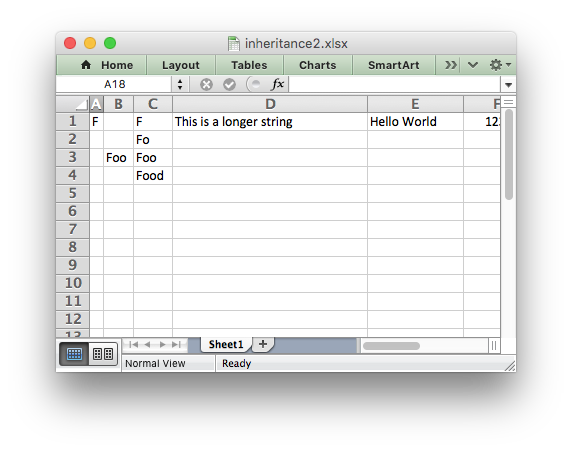# Example: Advanced example of subclassing#

Example of how to subclass the Workbook and Worksheet objects. See also the simpler inheritance1.py example.

In this example we see an approach to implementing a simulated autofit in a user application. This works by overriding the `write_string()` method to track the maximum width string in each column and then set the column widths when closing the workbook.

Some notes on this:

• This isn’t a fully functional autofit example (as shown by the longer strings in the screen shot). It is only a proof or concept or a framework to try out solutions. See the the worksheet `autofit()` method instead.

• The hard part is coming up with an accurate (or mainly accurate) `excel_string_width()` function. One possibility is to use the PIL ImageFont() method and convert the pixel width back to a character width.

• A more rigorous approach would have to consider font sizes, bold, italic, etc.

• The `set_column()` calls in `close()` will override any others set by the user. They also don’t set any column formats.

• It doesn’t work for horizontal merge ranges.

• There are probably some other corner cases hiding here.```##############################################################################
#
# Example of how to subclass the Workbook and Worksheet objects. See also the
# simpler inheritance1.py example.
#
# In this example we see an approach to implementing a simulated autofit in a
# user application. This works by overriding the write_string() method to
# track the maximum width string in each column and then set the column
# widths.
#
# Note: THIS ISN'T A FULLY FUNCTIONAL AUTOFIT EXAMPLE. It is only a proof or
# concept or a framework to try out solutions.
#
# Copyright 2013-2023, John McNamara, jmcnamara@cpan.org
#
from xlsxwriter.workbook import Workbook
from xlsxwriter.worksheet import Worksheet
from xlsxwriter.worksheet import convert_cell_args

def excel_string_width(str):
"""
Calculate the length of the string in Excel character units. This is only
an example and won't give accurate results. It will need to be replaced
by something more rigorous.

"""
string_width = len(str)

if string_width == 0:
return 0
else:
return string_width * 1.1

class MyWorksheet(Worksheet):
"""
Subclass of the XlsxWriter Worksheet class to override the default
write_string() method.

"""

@convert_cell_args
def write_string(self, row, col, string, cell_format=None):
# Overridden write_string() method to store the maximum string width
# seen in each column.

# Check that row and col are valid and store max and min values.
if self._check_dimensions(row, col):
return -1

# Set the min width for the cell. In some cases this might be the
# default width of 8.43. In this case we use 0 and adjust for all
# string widths.
min_width = 0

# Check if it the string is the largest we have seen for this column.
string_width = excel_string_width(string)
if string_width > min_width:
max_width = self.max_column_widths.get(col, min_width)
if string_width > max_width:
self.max_column_widths[col] = string_width

# Now call the parent version of write_string() as usual.
return super(MyWorksheet, self).write_string(row, col, string, cell_format)

class MyWorkbook(Workbook):
"""
Subclass of the XlsxWriter Workbook class to override the default
Worksheet class with our custom class.

"""

# Overwrite add_worksheet() to create a MyWorksheet object.
# Also add an Worksheet attribute to store the column widths.
worksheet.max_column_widths = {}

return worksheet

def close(self):
# We apply the stored column widths for each worksheet when we close
# the workbook. This will override any other set_column() values that
# may have been applied. This could be handled in the application code
for worksheet in self.worksheets():
for column, width in worksheet.max_column_widths.items():
worksheet.set_column(column, column, width)

return super(MyWorkbook, self).close()

# Create a new MyWorkbook object.
workbook = MyWorkbook("inheritance2.xlsx")

# The code from now on will be the same as a normal "Workbook" program.

# Write some data to test column fitting.
worksheet.write("A1", "F")

worksheet.write("B3", "Foo")

worksheet.write("C1", "F")
worksheet.write("C2", "Fo")
worksheet.write("C3", "Foo")
worksheet.write("C4", "Food")

worksheet.write("D1", "This is a longer string")

# Write a string in row-col notation.
worksheet.write(0, 4, "Hello World")

# Write a number.
worksheet.write(0, 5, 123456)

workbook.close()
```